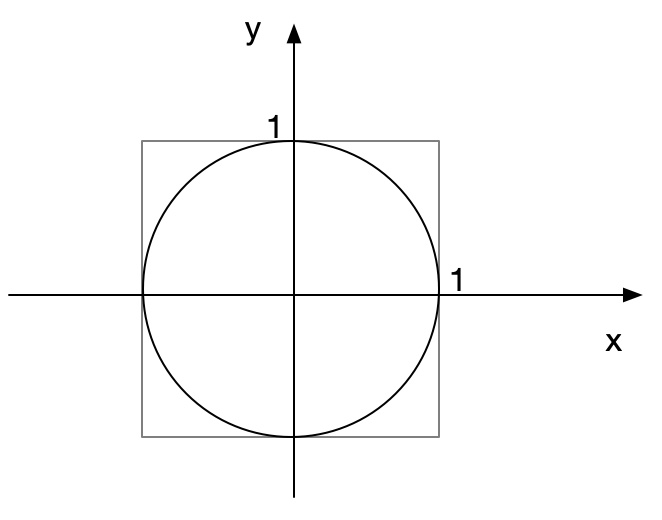# Estimate PI

Posted by chunyang on July 11, 2020

### Problem

The area of a circle is defined as $\pi r^{2}$ . Estimate $\pi$ to 3 decimal places using a Monte Carlo method.

Hint: The basic equation of a circle is $x^{2}+y^{2}=r^{2}$ .

### SolutionWe generate enough points in the up right of the rectangle. The probability is $\dfrac {\pi }{4}$ .

#include <iostream>
#include <iomanip>
#include <cstdlib>
#include <ctime>

using namespace std;

bool in_circle(double x, double y) {
return x*x + y*y <= 1;
}

double estimator_pi() {
const int COUNT = 3000000;
int number = 0;
srand(time(NULL));
for (int i = 0; i < COUNT; ++i) {
double x = rand()*1.0 / RAND_MAX;
double y = rand()*1.0 / RAND_MAX;
number += in_circle(x, y);
}
return 4.0 * number / COUNT;
}

int main() {

cout << setprecision(4) << "PI = " << estimator_pi() << endl;
return EXIT_SUCCESS;
}